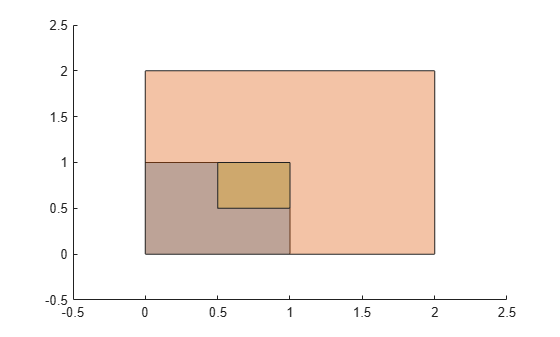# scale

Scale `polyshape`

## Syntax

``polyout = scale(polyin,s)``
``polyout = scale(polyin,s,refpoint)``

## Description

example

````polyout = scale(polyin,s)` returns a `polyshape` object defined by scaling `polyin` by a factor `s` with respect to the reference point (0,0). If `s` is a scalar, then `scale` applies it to both the x and y directions. If `s` is a two-element row vector, then the first element is the scale factor in the x direction and the second element is the scale factor in the y direction.```

example

````polyout = scale(polyin,s,refpoint)` specifies a reference point to scale with respect to.```

## Examples

collapse all

Create a polygon and scale it by a factor of 2.

```polyin = polyshape([0 0 1 1],[1 0 0 1]); poly1 = scale(polyin,2);```

Scale `polyin` by a factor of 0.5 with respect to the point (1,1). Plot all three polygons.

```poly2 = scale(polyin,0.5,[1 1]); plot(polyin) hold on plot(poly1) plot(poly2)```## Input Arguments

collapse all

Input `polyshape`, specified as a scalar, vector, matrix, or multidimensional array.

Data Types: `polyshape`

Scale factor, specified as a scalar or a two-element row vector. The elements of `s` must be positive.

• If `s` is a scalar, then `scale` applies it to both the x and y directions.

• If `s` is a two-element row vector, then the first element is the scale factor in the x direction and the second element is the scale factor in the y direction.

When the input `polyshape` is an array, `scale` applies `s` to each element of the array.

Data Types: `double` | `single` | `int8` | `int16` | `int32` | `int64` | `uint8` | `uint16` | `uint32` | `uint64`

Reference point, specified as a two-element row vector containing the x-coordinate and y-coordinate of the point to scale with respect to. When the input `polyshape` is an array, each element of the array is scaled with respect to `refpoint`.

Data Types: `double` | `single` | `int8` | `int16` | `int32` | `int64` | `uint8` | `uint16` | `uint32` | `uint64`

## Version History

Introduced in R2017b